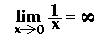QUESTION #251

# What is singularity and why do all laws of physics break down at singularity?

When a physicist refers to a singularity he or she is generally referring to a quantity which is infinite. Specifically, a quantity which approaches infinity as another parameter goes to zero, such asIt isn't true to say that all laws of physics break down at a singularity. You can imagine the problems though -- how do we interpret an infinite mass or infinite energy or infinite force? Usually we assume that there is some new set of laws or some new way of looking at the problem that makes the apparent singularity go away.

Here's an example. You may be familiar with Hooke's law for the force exerted by a spring: F=kx where k is the tension of the spring and x is the distance it is stretched. Now write the equation as k=F/x. Written this way it would seem that if you compare the tension between any two points on the spring, it grows and grows the closer together the two points are. In fact, two points spaced infinitesimally apart seem to have an infinite tension! It's just a manifestation of the 1/x limit above.

But of course that's not true. If you really want to know what's happening at small distance scales you can't use the classical physics behind Hooke's law. At some point x drops below the spacing between molecules in the spring's metal. Now Hooke's law no longer applies and you have to use atomic physics to explain the spring's properties. So in the large-scale theory (Hooke's law) there was no fundamental distance scale: x could be as small as you want. But at some point this law breaks down. In the small-scale theory (quantum mechanics and atomic physics) there is a fundamental distance scale: the atomic spacing. We would say that the singularity has been 'resolved.'

Most people worry about singularities involving general relativity: two examples being a black hole and the singularity that classical general relativity predicts was our universe at the moment it began. If you try to apply the laws of general relativity in these situations you will inevitably find the same 1/x singularities I've been talking about. How are we going to resolve these singularities? We expect quantum mechanics to do the job, since it is the theory that correctly describes physics at small distance scales. Unfortunately, while we have good theories of atomic physics, we don't real have a good theory of quantum gravity. Many of us think string theory will ultimately provide the resolution to these problems.

In short then, a singularity represents an infinity and we generally don't think nature is infinite. The problem arises from not having some kind of 'floor' built into a theory that keeps you from taking the limit of 1/x as x goes to zero. The way out is to apply a new theory that has such a floor, such as quantum mechanics or string theory (quantum gravity).
Answered by: Brent Nelson, M.A. Physics, Ph.D. Student, UC Berkeley